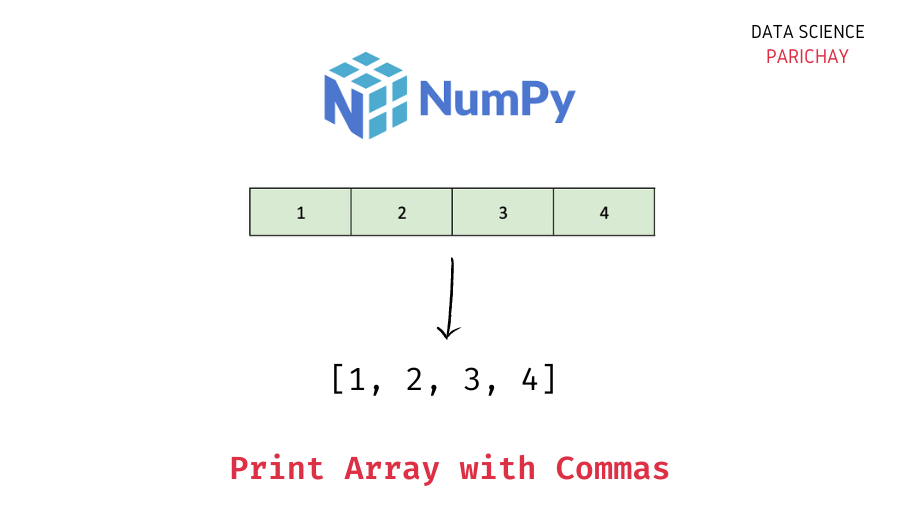# Numpy – Print Array With Commas

The Numpy library in Python comes with a number of useful functions to work with and manipulate arrays. In this tutorial, we will look at how to print a numpy array with elements separated by commas with the help of some examples.

## Printing a Numpy Array

First, let’s see what we get when we directly print a numpy array.

```import numpy as np

# create numpy array
ar = np.array([1, 2, 3, 4, 5])
# print the array
print(ar)```

Output:

`[1 2 3 4 5]`

Here, we created a numpy array of five numbers and then printed it using the `print()` function. You can see that the elements are printed with a space between them.

## How to print a numpy array with commas?

You can use the numpy `array2string()` function to print a numpy array with elements separated by commas. Pass the array and the separator you want to use (“,” in our case) to the `array2string()` function.

The following is the syntax –

```# print numpy array with "," separator
print(np.array2string(ar, separator=","))```

It returns a string representation of the array with the given separator.

Let’s now apply this function to the above array such that its elements are separated by commas on printing.

📚 Data Science Programs By Skill Level

Introductory

Intermediate ⭐⭐⭐

🔎 Find Data Science Programs 👨‍💻 111,889 already enrolled

Disclaimer: Data Science Parichay is reader supported. When you purchase a course through a link on this site, we may earn a small commission at no additional cost to you. Earned commissions help support this website and its team of writers.

```# print array with "," as separator
print(np.array2string(ar, separator=","))```

Output:

`[1,2,3,4,5]`

The array elements are separated by commas.

If the printed array looks cluttered, you can specify `, ` (a comma followed by a space) as the separator.

```# print array with ", " as separator
print(np.array2string(ar, separator=", "))```

Output:

`[1, 2, 3, 4, 5]`

The elements now look less cluttered.

### Use the `repr()` function

Alternatively, you can use the Python built-in `repr()` function to print a numpy array with commas. The `repr()` function is used to print the string representation of an object in Python.

Let’s look at an example.

`print(repr(ar))`

Output:

`array([1, 2, 3, 4, 5])`

The array elements are separated by commas. Note that the output is not exactly the same as what we got with the `array2string()` function.

## Summary – Print Numpy Array with Comma as a Separator

In this tutorial, we looked at how to print a numpy array with a comma as a separator. The following are the steps mentioned in this tutorial –

• Create a numpy array (skip this step if you already have a numpy array to operate on).
• Use the numpy `array2string()` function to get a string representation of the array with the desired separator (a comma in our case).
There are alternative methods as well, such as using the `repr()` function.

You might also be interested in –

•